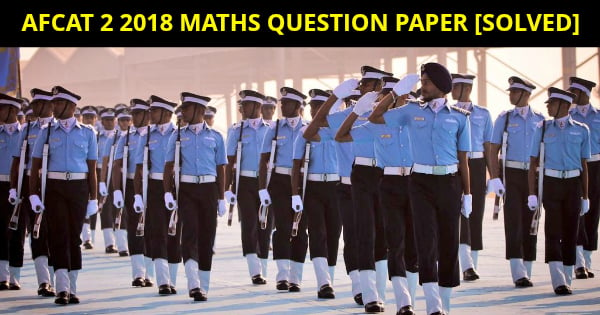# AFCAT 2 2018 Maths Question Paper [Solved]AFCAT 2 2018 Maths Question Paper: Here are some questions which candidates have faced in AFCAT 2 2018 online exam being conducted on 18th August 2018 and 19th August 2018. All the Maths numerical ability questions mentioned below are faced by the aspirants who took the AFCAT 2 2018  exam on 18th August 2018. We will be updating more AFCAT 2 2018 maths questions from both shift 1 and shift 2 of AFCAT 2 2018.
How To Prepare for SSB Interview:

#### AFCAT  Numerical Ability Topics

• Decimal Fraction
• Simplification
• Average
• Ratio and Proportion
• Simple interest
• Profit and loss
• Percentage
• Time and Distance### AFCAT 2 2018 Maths Question Paper

1. The difference between compound interest and simple interest in the 3rd year at a rate of 10% is 77.5 rupees is??
2. Two horses sold at 1200/-each . first one on a profit of 20% and second one is in 20%loss .the overall profit/loss in percentage is? – 4% loss
3. the intial price of a colour tv is three time of that music system ,now if price of tv is increase by 10% and music system by 18% ,then find the profit loss % on buying 8 colour tv and 5 music system?
4. The average of 5/16 and 3.6% is?
5. A and B make a 221 metre in 100/ 9 day . They both working on alternate days A starting the work make the same wall in 89/4 days . They both work together make a wall of double length to that wall and get 1800 rupees find the share of B in it??
6. Efficiency of A,B and C is in the ratio 4:5:6. What is the ratio of the time in which they complete the work?
7. Someone purchase egg in ₹100. 20% eggs break. Now on how much he should sold the remaining egg to get net profit of 10 %?
8. A bank give 16% interest per annum compounded semi annually. What interest a man get on amount of ₹10000 in 2 years?
9. If a stream takes 5 hours from A to B ….. And 3 hours from B to A …….if a wood log left at B and it start from B at 6:00 monday then at what time it will reach A?
10. 56^0.16×16^0.18=???
11. √ 0.0081+ √ .0064=?
12. If there is 25% increase in the cost of sugar by what % consumption should be decreased in order to maintain expenditure?
13. Average marks of a class are 70. if average marks of fail students are 40 and pass student is 80 marks. find % of pass students?
14. Population of a village increase 5% annually . if in2016 population 1852200 , find population in 2014?
15. If the difference between CI and SI is 77.5 Rs in 3yrs at 10% p.a . Ffind the sum ?
16. A alloy has element in 2:3 in how many step will A be more than 50% increasing 20% of A and 5% of B each time ?
17. Avg of 3.6 and 20% is?Download eBooks TAT eBook SRT eBook WAT eBook OIR Test NDA Solved Papers AFCAT Solved Papers Current Affairs eBook 101 Maths Shortcuts